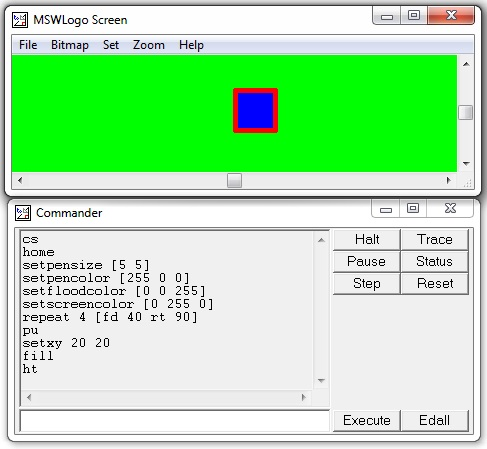# Logo - Introduction

Logo is a programming language that is very simple and easy to learn. It is used for teaching students and children how to program a computer.

## Why should we learn the Logo language?

• Because it is fun, lots of fun.
• Enhances the logical sense of the children.
• Develops programming skills.
• It is real Computer Science.

Logo is a very easy and interesting programming language to learn. It has enough depth to virtually do anything, which can be done in any other computer programming language.

If Logo Programming Software is not installed on the computer, we can get it for free from the following link −

www.softronix.com/mswlogo.html

After installation, it puts a Logo icon on the desktop of your computer.The MSW Logo screen has two parts −

• A Drawing window above with a triangle-shaped TURTLE in the center.
• A Commander window as shown in the following screenshot.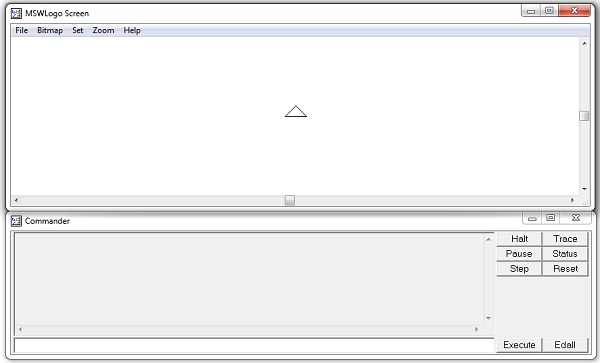We will write commands in the command line, i.e., the text box at the bottom of the Commander Window. To execute or run these commands, press Enter or click the Execute Button. We can also write and run more than one command online at a time.

The command history will appear in the gray box. Click a line in the history to make it jump to the respective command line, then we can make changes (if required). Once this is done, press Enter or click the Execute Button.

# Logo - Turtle

The simple Logo Drawing Commands move the Turtle forward and backward and also turn it right or left. The commands and their abbreviations are given below −

• fd – forward
• bk – backward
• rt – right
• lt – left
• cs – clearscreen

Either version of these commands can be used. Except the cs command, each of these commands must be followed by one value called as its argument. The arguments for fd and bk are units; those of rt and lt are angles that can be any integer. A rotation by 360 is a complete rotation, therefore a rotation by 375 degrees is the same as 1/15 degrees.

• forward 60 or fd 60 means go forward 60 steps

• right 90 or rt 90 means right turn 90 degrees

• left 90 or lt 90 means left turn 90 degrees

• back 60 or bk 60 means go back 60 steps

• clearscreen or cs means erase all drawings. This sets the turtle at the center

The graphics window has a coordinate system. The values of the two coordinates (normally called x and y) at the center are 0, 0. At the northeast corner, they are 250, 250; at the southeast corner, they are 250, -250. At the southwest corner, they are -250, -250; etc. If the turtle tries to walk off onto one side of the screen, it wraps around. The right side wraps to the left side and the top wraps to the bottom.

Many programming systems work on the same kind of two-axis ‘xy’ coordinate plane, which we work with in Algebra as well.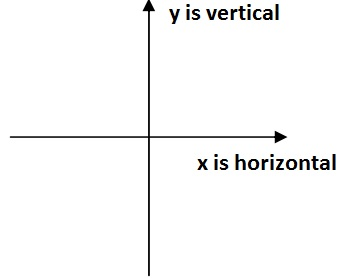Here, ‘0 0’ is the center, or origin (no comma or parentheses here!). In its centered, zoom-"normal" state, Logo's drawing screen shows an area of about 150 points up or down and 300 points right or left from the center.

The turtle can be directed with headings that correspond to a compass rose, with 0 or 360 degrees pointing straight up, 90 degrees straight to the right, and so on. You can set a variable to a number between 0 and 360 and then walk on that path.

## Turtle Commands

Now let us try some commands. Commands will be issued one per line followed by a carriage return. Several of these commands can be typed in succession in a command window followed by a carriage return. The effect on the turtle is the same. However, if you type a command, which requires one or more inputs and provide the missing input(s) on the next line, Logo will show an error.

Following is a practice command, which shows the desired results on the right.The commands – fd 50 rt 120 fd 50 rt 120 fd 50 rt 120, cause the turtle to draw a triangle, as you can see by trying them out.

These commands are read from the left to the right. Since the command fd requires one argument, it is taken as the next value. Similarly, rt takes an argument as well. Thus, Logo can give an unambiguous meaning to each of these character strings. For some Logo commands, separators are needed.

Following are few practice commands with the desired results on the right.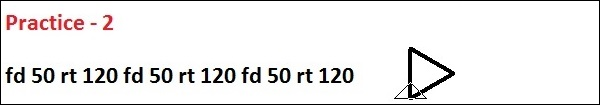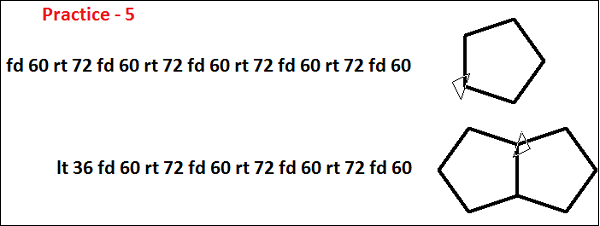Following is an exercise to check your aptitude on what you have learned so far in this chapter.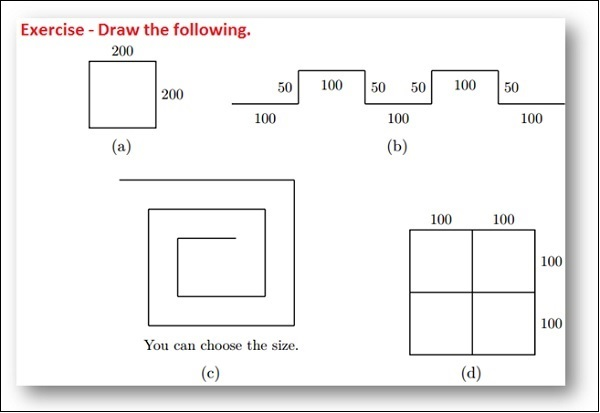# Logo - Controlling the Turtle & Pen

Logo has a number of other drawing commands, some of which are given below.

• pu − penup

• pd − pendown

• ht − hideturtle

• dt − showturtle

• setpensize

The pendown and penup commands tell the turtle to leave ink on the screen as it moves or not to leave ink, respectively. The hideturtle and showturtle commands hide or show the turtle, but do not affect its ability to leave ink as it moves. The home command causes the turtle to return to the center of the screen. It may leave ink behind, when the turtle returns to the center of the screen. The setpensize command decides the drawing pen size.

• penup or pu means pick pen up, so you can move turtle without leaving tracks.

• pendown or pd means pick pen down, so you can move the turtle and leave tracks.
• hideturtle or ht means hide the turtle, so you can admire your drawing.

• showturtle or st means show the turtle, so you can continue your drawing.

• setpensize means it can make the pen larger, easier to see. Default pen size is –[1 1].

Following are few practice commands with the desired results on the right.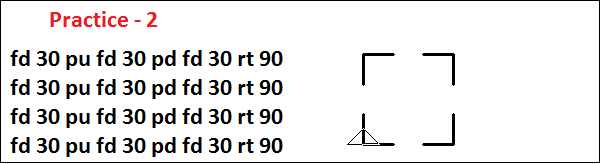# Logo - Turtle World

Logo has a number of other additional drawing commands, some of these are given below.

• home
• cleartext or ct
• label
• setxy

The label command takes a single word as a quoted string (e.g. “a_string”) or a list of words in [ ] brackets without quotation (e.g. [a string of letters]) and prints them on the graphics window at the location of the turtle. Let us consider the following code.The setxy command takes two arguments, treats the first as the value of the abscissa (horizontal axis) and the second as a value of the ordinate (vertical axis). It places the turtle at these coordinates, possibly leaving ink while reaching these coordinates. In the following three figures, we have shown how the setxy command can be used.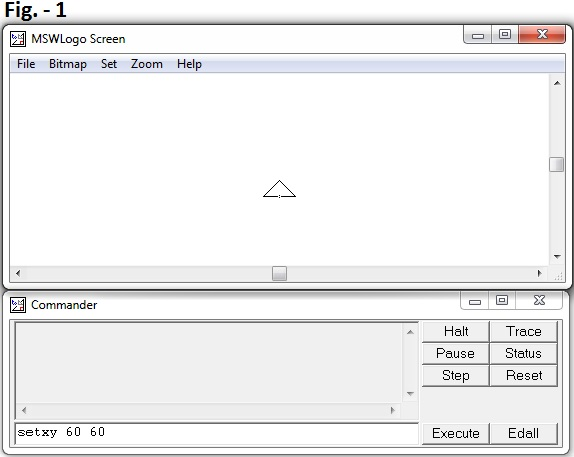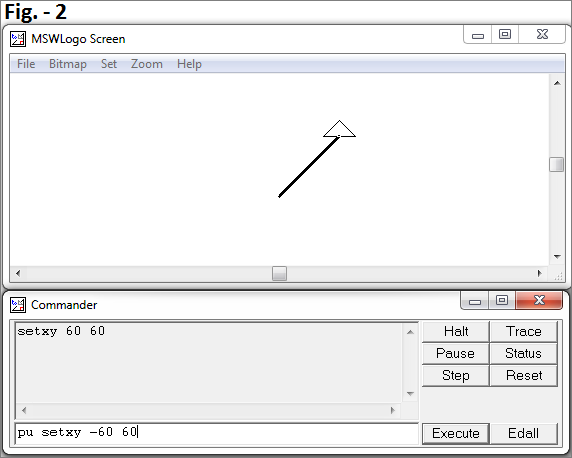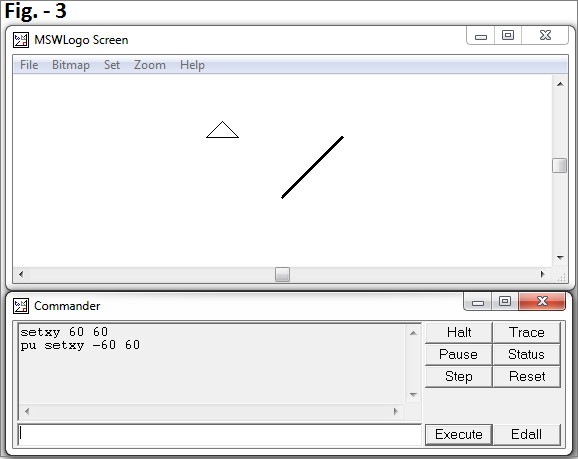The cleartext command, abbreviated ct, clears the text region of the command window.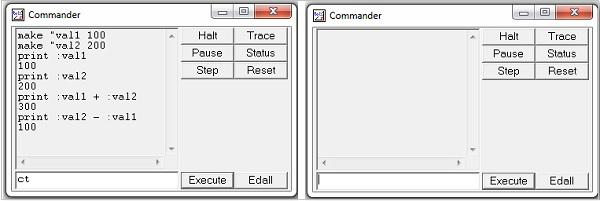## Exercise

Following is an exercise to check your aptitude on what you have learned so far in this chapter.

What kind of figure does the following command sequence produce?

```cs pu setxy -60 60 pd home rt 45 fd 85 lt 135 fd 120
```

Interpret these commands as you read them from left to right. Try it to find out the result.

Following is a table of command summary.

Command Name Purpose
setx 100

Sets the turtle's x-coordinate to +100

Moves it 100 points to the right of center

No vertical change

setx -200

Moves the turtle 200 points to the left of center

No vertical change

sety 150

Sets the turtle's y-coordinate to 150

Moves it 150 points above center

No horizontal change

sety - 50

Moves the turtle 50 points below center

No horizontal change

setxy 100 100

Moves the turtle to xy coordinate 100 100

show xcor

show ycor

Reports the turtle’s x-coordinate

Reports the turtle’s y-coordinate

seth 0

Points the turtle straight up, “high noon”

seth 120

Moves the turtle 120 degree to point to the four o’clock position

The following screenshot is a practical demonstration of some of the above commands.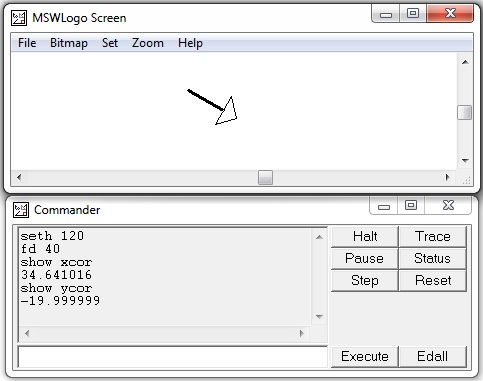# Logo - Variables

A variable is the name of a memory location which can contain a value. In a computer, each memory location has an integer address. Since it would be hard to remember the address of each location containing a value used by a program, computer scientists have found ways of giving these locations, symbolic names. Once a variable has a name, we can use and manipulate it.

Variables are given names which are strings of letters. A variable name can contain alphabets (case insensitive), digits and underscore. A variable name can be accessed in a computation using ‘:’ before it. Let us consider the following example in the screenshot.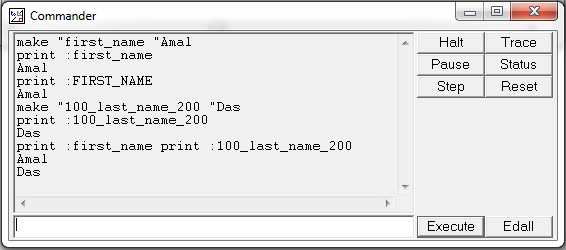In the above example, we have defined two variables first_name, 100_last_name_200 and initialized them with values Amal and Das using the following statements −

• make “first_name “Amal
• make “100_last_name_200 “Das

Also, we printed these two variables with statements print :first_name and print :100_last_name_200.

The following example shows how to define numeric variables −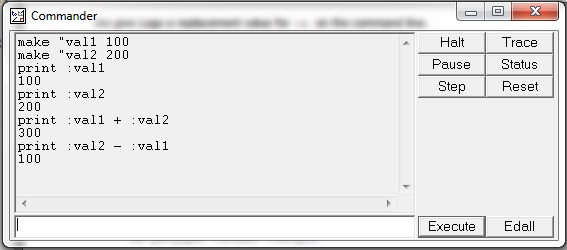Here, we have defined two numeric variables val1 and val2. We have also performed addition and subtraction using them.

# Logo - Arithmetic Operations

Logo provides the usual arithmetic operations of addition, subtraction, multiplication and division, denoted by the symbols +, -, *, /. Each of these operations produces a result. If you don't do something with the result, such as print it, Logo will show an error.

With the print command, the result of an arithmetic operation can be used and printed in the command window. Examples given in the following screenshot demonstrate the same.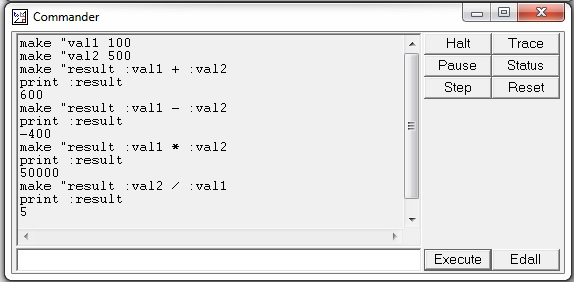Other useful commands are −

• sqrt − It takes one non-negative argument and returns its square root.

• power − It takes two arguments, call them ‘a’ and ‘b’, and generates a to the b power.

• ln − It takes one argument and returns its natural logarithm.

• exp − It takes one argument and computes e to that power, e is the natural number 2.718281828.

• log10 − It takes the logarithm to base 10 of its one argument.

Following screenshot shows an example of the above commands with their respective output.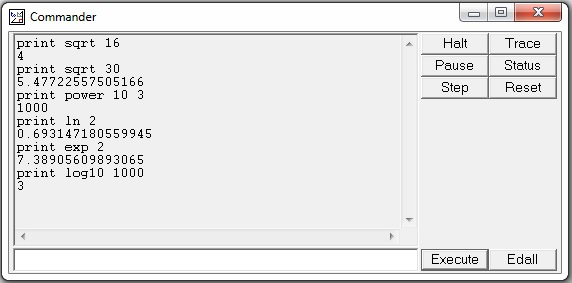Arithmetic operators have a precedence that determines the order with which they are evaluated.

Note − print 60 * sqrt 2 and print sqrt 2 * 60 produce different answers. Here the * operator has a precedence over the sqrt operator. Thus, * will be done before sqrt, if there is a choice, as there is in the second case.

For this reason, the first statement prints the value of 60 times the square root of 2, whereas the second statement prints the square root of 120 as shown in the following screenshot.# Logo - Repetition

We often repeat a sequence of commands. Computer programs often perform repetitive tasks. Just about every programming system has a way of carrying out this repetition, or iteration, as computer scientists call it. Let us consider the following example −

Let us assume we want to draw a square with sides of length 100, we can do this with the following program −

```fd 100
rt 90
fd 100
rt 90
fd 100
rt 90
fd 100
rt 90
```

We note that the two commands – fd 100 and rt 90 are repeated four times. Will it not be simpler to tell the computer that it should just repeat these two commands four times instead of writing them four times in a row? We can do exactly this, using the following command −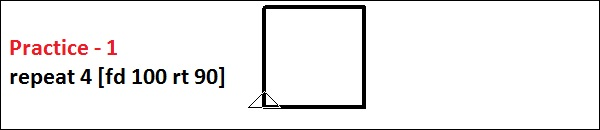It saves our time of typing-in to make a square. The general form is: repeat number [commands]. We must use the keyword – repeat followed by a number and then a sequence of commands in [square brackets].

Often, we might have to repeat within repeat. This is called nesting. Let us look at some examples on this.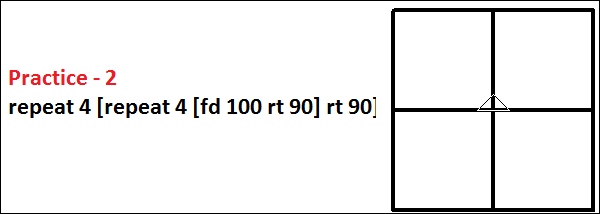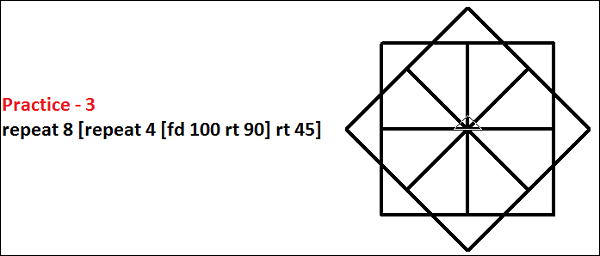Following is an exercise to check your aptitude on what you have learnt so far in this chapter.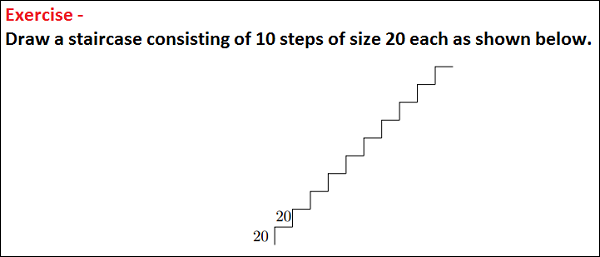# Logo - Randomization

Sometimes it is fun to have an unpredictable outcome of a computation. Logo provides a random procedure to generate a random number. It has one argument and produces an integer value chosen uniformly at random, which is greater than or equal to 0 and less than the value of its argument. Thus, if you want a random angle between 0 and 359 degrees, you can use the command random 360 to produce it. Bear in mind that Logo will show an error unless you do something with the result, such as printing.

Let us look at the following example −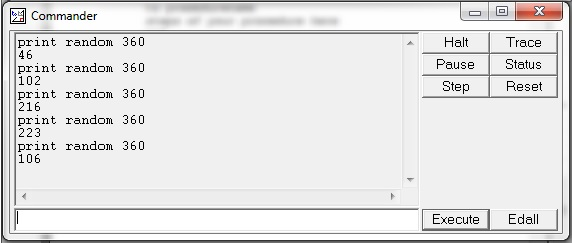We have issued the command – print random 360 several times in the above command window and if you notice, each time it generates a random output.

Following are few practice commands with the desired results on the right.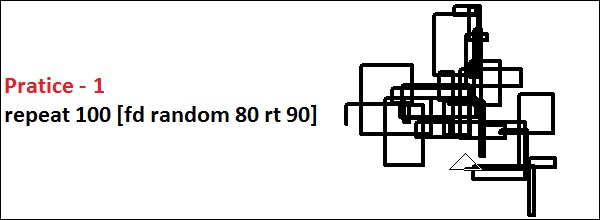# Logo - Procedures

Procedures provide a way to encapsulate a collection of commands. Once a procedure has been created, it can be used just the way a built-in command is used. The “meaning” of a procedure is the meaning of its individual commands.

A procedure without arguments has the word ‘to’ (a reserved word) and the name of the procedure on the first line. (Reserved words in Logo cannot be used as variables and have a well-defined meaning and use.) It has the reserved word ‘end’ on the last line.

A subprogram is a named sequence of steps for another program to execute. Other names for subprograms are procedures and functions. In Logo, you tell the computer how to do something — for example −

```to square
repeat 4 [fd 100 rt 90]
end
```

Once we have described our procedure to Logo, we can enter its name on the command line, just as we would do to any of the built-in things. In this case, we would type ‘square’ on the command line and Logo looks up the commands to make a square.

Click the button that says Edall (for edit all) to bring up Logo's built-in editor. (If your Logo doesn't have an Edall button, type ‘edall’ on the command line). The following code block has the required structure of the subprogram.

```to procedurename
end
```

The procedure or subprogram must start with the word ‘to’, followed by a name we think of. The next step is to key-in all the same steps we would write on the command line. The procedure must end with the word ‘end’. All comment or remark lines should be preceded by semi-colon (;).

Following is the practical demonstration of the above example −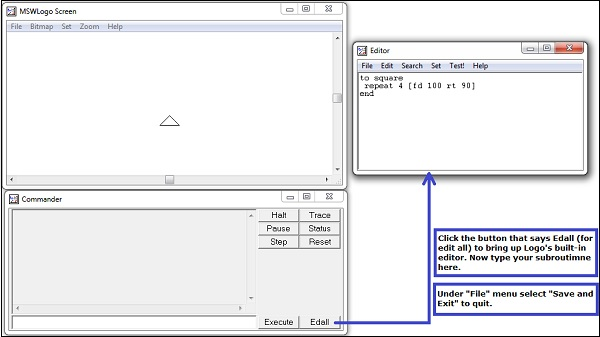Now, from the command line, execute the procedure using its name “square” as shown below −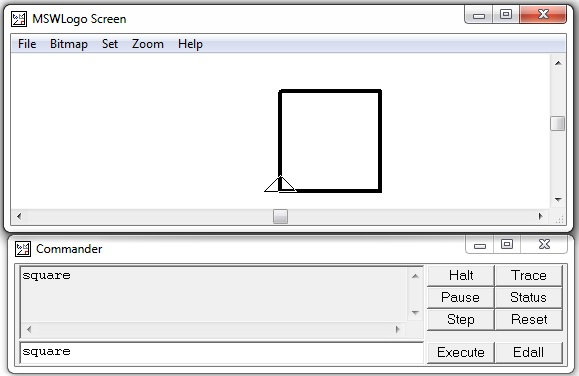Procedures can not only contain built-in commands, but they can also contain other procedures.

In the following example, a procedure ‘flower’ is calling our predefined procedure ‘square’ from its body.Following screenshot shows the output when the procedure “flower” is called −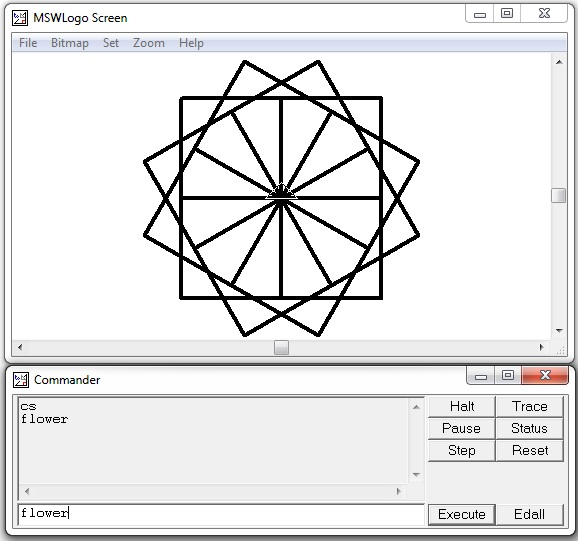We don't want every square to be of the same size — we want variety. In Logo, we create variables, whose values we can change. We will use the same square procedure with a small change in the following example.

```to square :n
repeat 4 [fd :n rt 90]
end
```

We give Logo a replacement value for ‘:n’ on the command line as shown below.

```square 50
square 75
square 100
```

Here is the practical demonstration of the above example −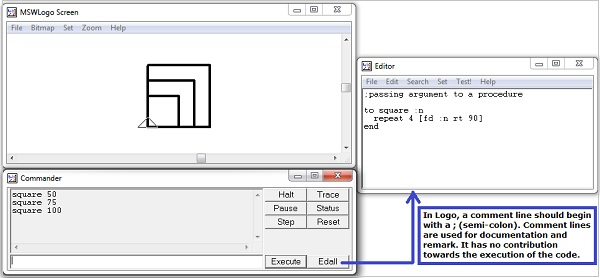Now let us discuss how to pass two arguments to a procedure. Following screenshot is a practical demonstration of the same.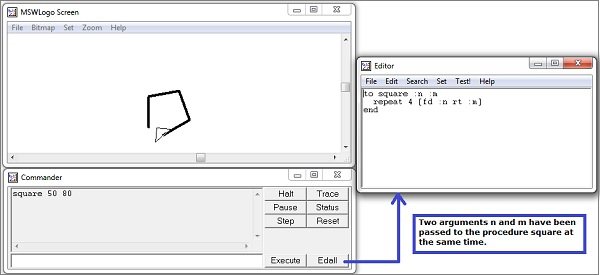# Logo - Recursive Procedures

In a recursive procedure, there will be a recurrence call of the procedure within the procedure. Let us consider the following code −

```to spiral_recur :n
if :n < 1 [stop]
fd :n
rt 20
spiral_recur 0.95 * :n
end
```

The procedure spiral_recur has been called from the procedure body itself. The following screenshot shows the execution and output of the code.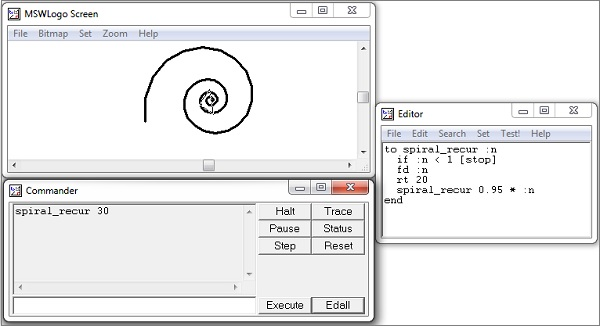# Logo - Decision-Making

Decision-making and variables go together. A program needs to be able to change course depending on the situation. Here, for example, is a framework for drawing a spiral. It has a loop, a variation on the repetition shown earlier and the body of the loop is for us to fill in.

```to spiral
make "n 1
while [:n < 100] [
make "n :n + 5
fd :n rt 90
]
end
```

The above code shows several new features of the syntax of the MSW Logo. We set a variable to a new value by keying-in ‘make’, then the variable's name is preceded by a double quote " rather than a colon ‘:’ as shown below.

```make "n 1
```

We use a variable, though, with a colon ‘:’ in front of its name.

```while [:n < 100]
```

The code bracketed after the ‘while [condition]’ is executed, while the condition is true. When it is no longer true, because (in this case) the value of ‘:n’ grows greater than 100, the code following the bracket is executed.

Following screenshot shows the execution and output of the above code.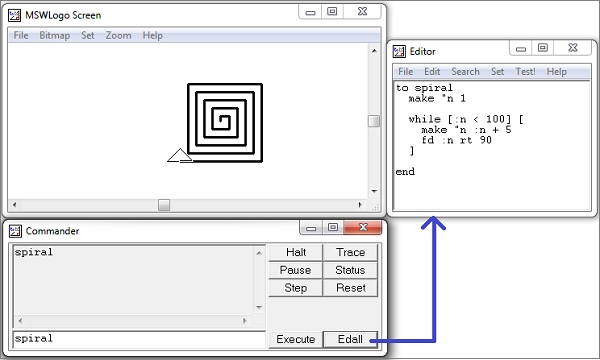Now, we shall discuss the use of ‘if statements’, which have a code that will be executed only when a given condition is true.

It also shows a built-in Logo that generates random numbers. The statement random 3 generates any number 0 or 1 or 2 arbitrarily in a random sequence. The procedure then decides which way to go "at random". The generated random number will be kept in ‘r’ and later depending upon the value of the variable ‘r’ one of the if-statements will get executed, which will satisfy the condition. Thus if the −

• Value of ‘r’ is 0, then [fd 20] will be executed.
• Value of ‘r’ is 1, then [rt 90 fd 20] will be executed.
• Value of ‘r’ is 2, then [lt 90 fd 20] will be executed.

Following screenshot shows the execution and output of the above discussion.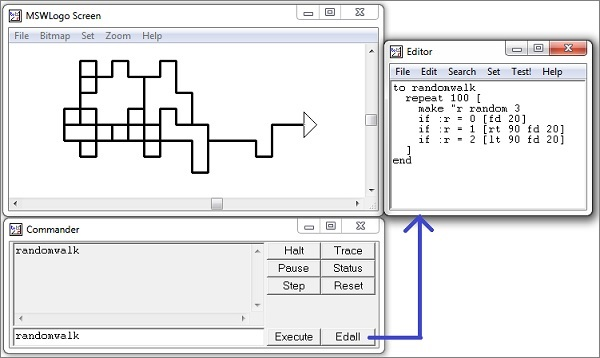# Logo - Strings

Any sequence of alpha-numeric characters, for example – “america”, “emp1234”, etc. are examples of a string. Counting the characters is the most basic of all string processes. The answer to the question stringlength "abc12ef is given by the following procedure −

```to stringlength :s
make "inputstring :s
make "count 0
while [not emptyp :s] [
make "count :count + 1
print first :s
make "s butfirst :s
]
print (sentence :inputstring "has :count "letters)
end
```

In the above procedure –‘s’ is the variable containing the input string. Variable inputstring contains the copy of the input string. Variable count is initialized with 0. In the while loop, the condition checks whether the string has become empty or not. In each loop count, a variable is being increased by 1 to hold the length count. The statement print first :s, prints the first character only of the string stored in ‘s’.

The statement make "s butfirst :s, retrieves the sub-string excluding the first character. After exiting from the while-loop, we have printed the character count or the length of the input string. Following is the execution and output of the code.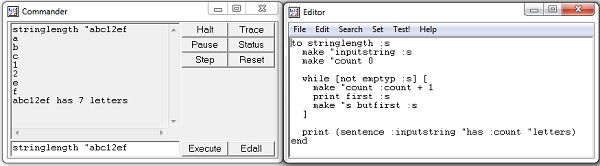# Logo - Color

Computer screens work with red, green and blue components of light, so they are sometimes called RGB screens.

On Logo's Set menu, we can set the color of three screen elements −

• The turtle's pen
• The turtle's fill (like a paint bucket for enclosures)
• The screen background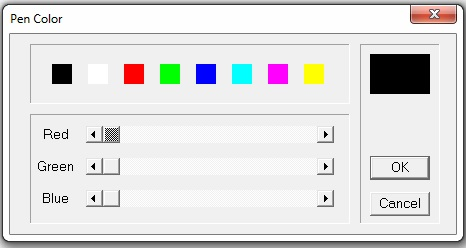We set a color by moving these three sliders left and right. Remember that black is the absence of all color and white is all colors together. Mixing light isn't like mixing paint. As an example, if you mix red and green paint, you get a muddy color. Since this is a computer, every color has an internal numeric representation.

On the left end of the sliding scale is zero (0). On the right end is 255, which is kind of like 99 to a computer (It's 28 - 1). Thus black is [0 0 0], red is [255 0 0], green is [0 255 0], blue is [0 0 255]. You can make anything in between these colors, and in all these, there are 256 * 256 * 256 possible colors. That's 28 * 28 * 28, or 24-bits of color — 24-binary digits inside the machine.

The following commands will give you a big fat red pen −

```setpensize [5 5]
setpencolor [255 0 0]
```

When you find a color you like using the sliders, you can ask Logo what it is: choose the pen color, then in the command window, enter the following command.

```show pencolor
```

The following screenshot shows the execution and output of the above code.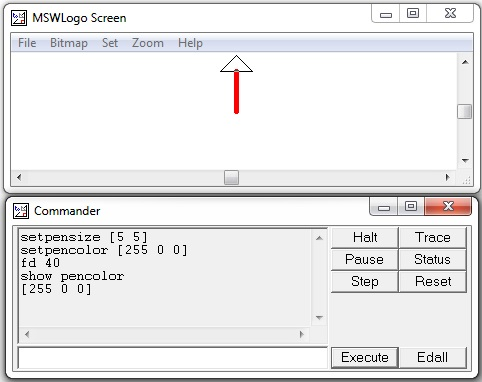You can make a colored square using the following steps −

Step 1 − Draw the square with side length 40 using the following command.

```repeat 4 [fd 40 rt 90]
```

Step 2 − Pen up using the following command.

```pu
```

Step 3 − Go to a point inside the square. For example, place the turtle at coordinate (20, 20) using the following command.

```setxy 20 20
```

Step 4 − Fill the square with the set floodcolor. For example, to set the floodcolor to blue use the following command.

```setfloodcolor [0 0 255]
```

The following table lists a few more Color and pen related commands.

Color & Pen command Purpose of the command

setpencolor [ r g b]

setpc [r g b]

Sets the color for turtle’s pen

r g b are numbers in range [0, 255]

setfloodcolor [r g b]

setfc [r g b]

Sets the color for an endorsed area

setscreencolor [r g b]

setsc [r g b]

Sets the color for the background

show pencolor

show floodcolor

show screencolor

Specifies the current values for [r g b] of a named item

Fill

Dumps a bucket of current floodcolor at the cursor’s location

Fill

Dumps a bucket of current floodcolor at the cursor’s location

Try executing the following set of commands −

• cs − To clear the screen.

• home − To bring the turtle at the home place.

• setpensize [5 5] − Setting the pen size.

• setpencolor [255 0 0] − Setting the pen color to red.

• setfloodcolor [0 0 255] − Setting the flood color to blue.

• setscreencolor [0 255 0] − Setting the screen color to green.

• repeat 4 [fd 40 rt 90] − Draw a square with side length 40.

• pu − Pen up.

• setxy 20 20 − Put the turtle at coordinate (20, 20).

• fill − Fill the square with the set floodcolor blue.

• ht − Hide turtle.

You should receive the following output on execution of the above commands.